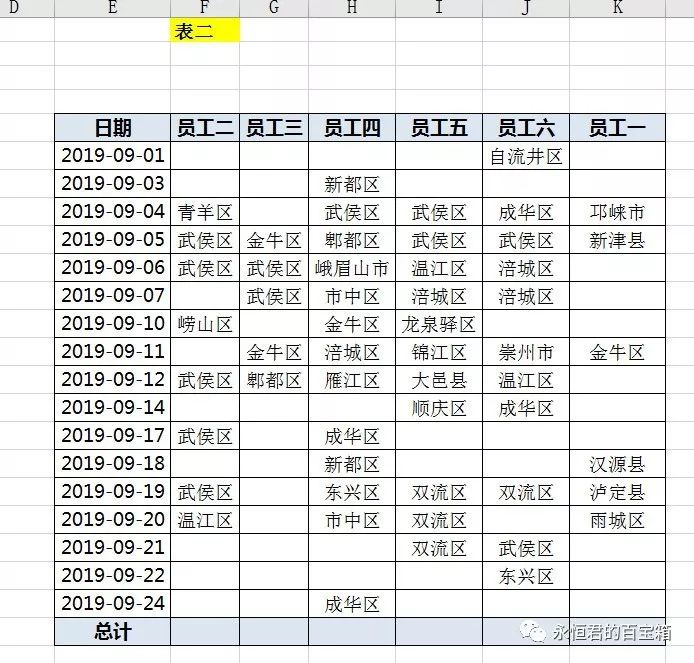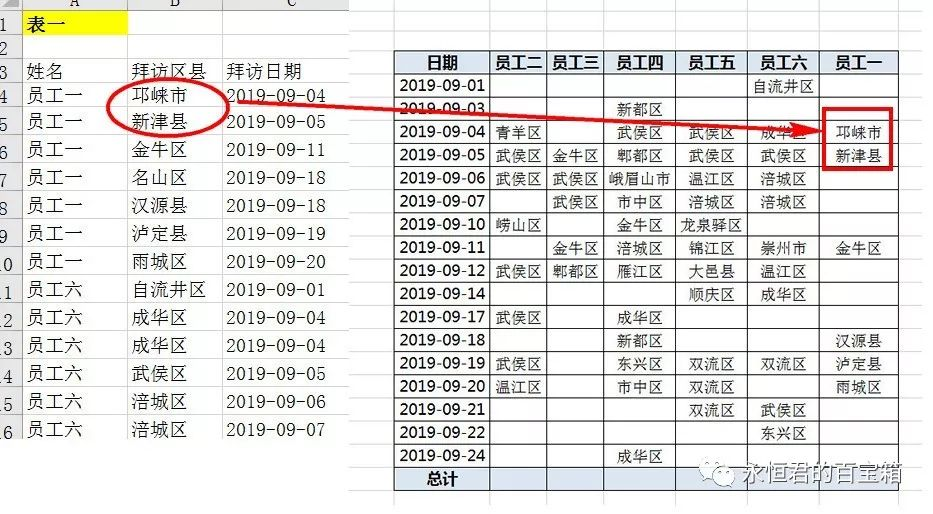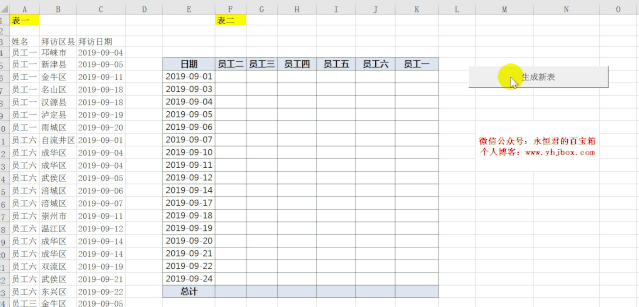﻿﻿ Excel vba 实例(20) – 一键填充每月员工拜访地区 - 永恒君的百宝箱

# Excel vba 实例(20) – 一键填充每月员工拜访地区

275℃#### 思路#### 核心代码

``````For i = 6 To 22 '遍历表二每一列
For j = 6 To 11 '遍历表二每一行
For k = 4 To 63 '遍历表一数据每一行
If Cells(k, 1) = Cells(5, j) And Cells(k, 3) = Cells(i, 5) Then '做逻辑判断
Cells(i, j) = Cells(k, 2)
End If
Next
Next
Next
``````Excel vba 实例(19) –  一键汇总不完全相同的sheet到总表Excel vba 实例(18) – 一键将单列长数据平均拆成多列

Excel vba 实例(17) – 遍历多个工作簿并提取内容到总表

Excel vba 实例(16) – 按指定字段分类批量提取内容anyShare分享到：